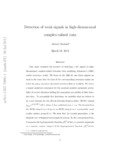## Detection of weak signals in high-dimensional complex-valued data

Onatski, A
##### DOIArticle
##### Abstract

This paper considers the problem of detecting a few signals in high-dimensional complex-valued Gaussian data satisfying Johnstone's (2001) \textit{spiked covariance model}. We focus on the difficult case where signals are weak in the sense that the sizes of the corresponding covariance spikes are below the \textit{phase transition threshold} studied in Baik et al (2005). We derive a simple analytical expression for the maximal possible asymptotic probability of correct detection holding the asymptotic probability of false detection fixed. To accomplish this derivation, we establish what we believe to be a new formula for the \textit{% Harish-Chandra/Itzykson-Zuber (HCIZ) integral} $\int_{\mathcal{U}(p)}e^{\tr(AGBG^{-1})}dG$, where A has a deficient rank r<p. The formula links the HCIZ integral over U(p) to an HCIZ integral over a potentially much smaller unitary group $\mathcal{U}(r)$. We show that the formula generalizes to the integrals over orthogonal and symplectic groups. In the most general form, it expresses the hypergeometric function 0F0(α)of two p×p matrix arguments as a repeated contour integral of the hypergeometric function 0F0(α)of two r×r matrix arguments.

2014
2014-03-19
2013-12-19
##### Keywords
Spiked covariance, sub-critical regime, signal detection, sphericity tests, asymptotic power, contiguity, power envelope, Harish-Chandra/Itzykson-Zuber integral, torus scalar product, hypergeometric function
##### Journal Title
Random Matrices: Theory and Application
2010-3263
2010-3271
3
##### Publisher
World Scientific Pub Co Pte Lt